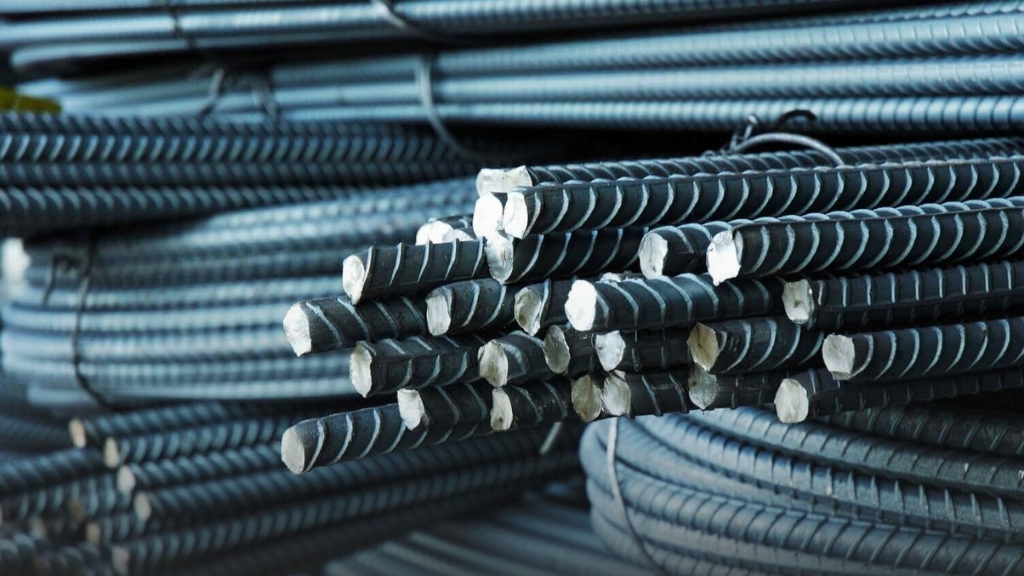# What is Unit Weight of Steel Bars & How to Calculate it?## Unit Weight of Steel: Definition and Calculation

Steel is a ductile material commonly found on construction sites, and engineers need to determine the unit weight of steel in their designs. So what is steel? Steel is iron with the addition of carbon and other metallic elements in small amounts. These additional materials change the properties of steel. Steel is magnetic just like cobalt, nickel, and iron.

## What is The Unit Weight of Steel?

The weight of steel is calculated as follows

Weight = Density x Volume

The unit weight of steel density is also defined as the ratio of its mass to volume. Specifically, it is 7850 kg/m3 or 78.5 kN/m3, or 8050 kg/m3 or 80.5 kN/m3.

The density of steel ranges from 8050 kg/m3 to 7850 kg/m3, depending on the type and grade of manufacture.

However, the unit weight of steel in design standard EN 1991-1-1 Table A.4 is commonly used as 78.5 kN/m3 with a bulk density of 7850 kg/m3. It is also used by civil engineers in other countries as a density for steel.

The most common diameters of steel bars in the construction industry are 40mm, 32mm, 25mm,  20mm, 16mm, 12mm, 10mm, 8mm, and 6mm.

## Weight of Steel Bars

 Bar size diameter (mm) Perimeter (mm) Weight of Bar Kg/ft Kg/m 6 18.85 0.728 0.222 8 25.1 1.296 0.395 10 31.4 2.031 0.619 12 37.7 2.913 0.888 16 50.2 5.180 1.579 20 62.8 8.100 2.469 25 78.5 4.554 1.388 32 100.5 20.712 6.313 40 125.6 32.362 9.864

## How to Calculate the Unit Weight of Steel: Steel Weight Formula

The density of steel = 7850 kg/㎥

Weight of steel per meter = density x volume

Where, volume = length x area

Where, length = 1000 mm

And, area = π/4 x d2

Therefore, the weight of steel per meter = 1000 x π/4 x d2 × 7850 kg/m3

= 0.785 × 1000 × d2 × 7850 kg / (1000 × 1000 × 1000) (meter = 1000 mm)

= 785 × 0.00000785 x d2

= d2/162 kg/m

Now let’s understand calculating the unit weight of steel better by taking two examples of 8 mm and 16 mm steel bars.

## Steel Weight Per Meter of 8 mm Bars

Number of steel bars = 5

Diameter of the steel bar =  8 mm

Length of the bar = 1 m

Density of steel = 7850 kg/m3

Therefore,

Area of circular bar = π/4 x d2 = π/4 x 8^2 = 50.27 mm2

We know,

Volume = area x length = 50.27 x 1000 = 50270 mm3

Therefore, the steel weight per meter of a 8 mm bar = 50270/(10^9) x 7850 = 0.395 kg/m

Note: To convert mm3 to m3, multiply it by 1 x 10^9 (1,000,000,000)

## Steel Weight Per Meter of 16 mm Bars

Number of steel bars = 5

Diameter of the steel bar =  16 mm

Length of the bar = 1 m

Density of steel = 7850 kg/m3

Therefore,

Area of circular bar = π/4 x d2 = π/4 x 16^2 = 201.06 mm2

We know,

Volume = area x length = 201.06 x 1000 = 201061 mm3

Therefore,

The steel weight per meter of a 16 mm steel bar = 201061 /(10^9) x 7850 = 1.578 kg/m

Note: To convert mm3 to m3, multiply it by 1 x 109 (1,000,000,000)

## A Few Words About The Uses of Steel Bars

Steel bars are a commonly found in reinforced concrete, that takes the tensile stresses in concrete because concrete is not good in handling tension loads, unlike compressive loads. Reinforced concrete and steel bars are used in the construction of slabs, columns, dams, beams, and other such structures.

The density of concrete with no steel bars is 2400 kg/m3. But when we calculate the unit weight of steel in concrete, we increase the density of concrete to 2500 kg/m3 to take into account the steel bar weight.

## A Final Word: What is The Yield Stress of Steel bars?

Yield stress or YS is the stress level at which steel bars begin to permanently deform. At this point, the bars cannot retune to their original size or shape even if the applied stress is released. Usually, YS is 500 N/mm2. To determine the yield strength of steel bars, you must refer to manufacturers and national technical standards. The yield strength of concrete steel bars ranges from 400 to 600 MPa (N/mm2).

### 1. What is the unit weight of steel in kN m3?

The unit weight of steel is the ratio of the weight of steel to its volume. By that calculation, the unit weight of steel is around 78.5kN/m3 or 7850kg/m3.

### 2. How do you calculate the unit weight of steel?

The formula for calculating the weight of a steel bar is W=D2L/162.

### 3. What is the weight of steel in kg?

The unit weight of steel in kg is 7850 kg/m³.#### Property Geek

Share now!Here's more to look into!ENQUIRY